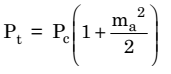# Power of A Single-Tone Amplitude Modulated (AM) Signal

## Power of A Single-Tone Amplitude Modulated (AM) Signal

### Mathematical Expressions

Let us consider that a carrier signal A cosωct is amplitude-modulated by a single-tone modulating signal

x(t) = Vmcosωmt.

Then the unmodulated or carrier power is :

Pc = mean square value of A cosωct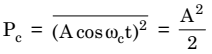The sideband power is given by :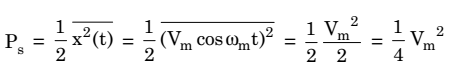We know that the total modulated power Pis the sum of Pc and Ps. Therefore ,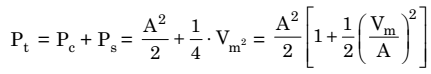But,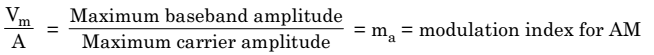Hence,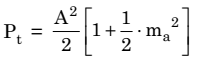But,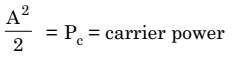Therefore,